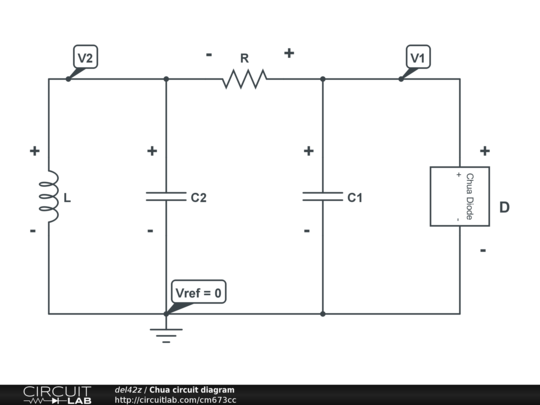# circuit equations derivation problem

I'm trying to derive from scratch the differential equations that characterise Chua's circuit.Here are the ODEs I obtain from that diagram. Current is positive if conventional current goes + → –.

equations http://imageshack.us/a/img822/8892/diffeqwithdim.png

This thing is, wherever I look, the IL term in the dV2/dt equation is positive instead of negative, with everything else effectively the same. (Also, beta is generally positive).

Why am I getting the sign of that IL term wrong?

• Can't see the equations ... seems to be an imageshack problem. – Dave Tweed Nov 3 '12 at 11:47

## 1 Answer

Why am I getting the sign of that IL term wrong?

The voltage across an inductor is related to the current through the inductor as follows:

$v_L = L \dfrac{di_L}{dt}$

where the reference direction for the current is into the positive labelled terminal.Since:

$v_L = V_2$

it immediately follows that

$\dfrac{di_L}{dt} = \dfrac{V_2}{L}$

• That would solve the problem, but why is it that most sources used -L instead of L in that first equation? – del42z Nov 4 '12 at 20:16
• @del42z, in circuit theory, you'll only find the equation I give. That's because of the so-called passive sign convention (PSC). In physics, you'll often find the equation for the emf of an inductor and that has the opposite sign. For example, look at the last equation on this page: faculty.wwu.edu/vawter/PhysicsNet/Topics/Inductance/… The key thing to note is that, using a voltmeter, you'll measure the voltage across the inductor, not the emf. – Alfred Centauri Nov 4 '12 at 20:54
• Ok, that makes sense, as the voltage has to balance the emf of the inductor, and that voltage is what is measured. Thanks. – del42z Nov 4 '12 at 21:06